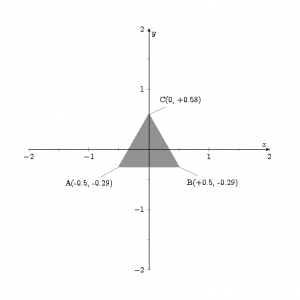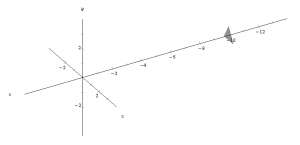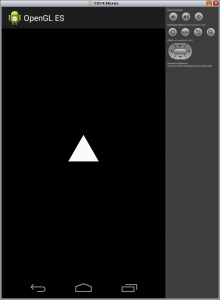# Drawing a triangle with OpenGL ES in Android

The scope of this post is drawing a triangle with OpenGL ES 1.x in Android focusing especially on the relationship between code and position of the triangle.
The coordinate system has the origin where the observer is, with the x axis horizontal and rightward, the y-axis vertical and upward and the z axis points so that the observer is looking towards the negative z, then the coordinate system follows the right-hand rule.
The 3 vertices of the triangle have coordinates:

• A(-0.5, -0.29, -10)
• B(+0.5, -0.29, -10)
• C(0, +0.58, -10)

that are the vertices of an equilateral triangle with the barycenter in (0, 0, -10).

In our view the triangle appears as in figure:If we go out from our perspective the scenario appears as in the figure below where the observer is located at the origin of the axesTo draw the triangle with OpenGL ES 1.x in Android follow these steps:

1. create an Android project without initial activity
2. edit the file AndroidManifest.xml
3. create the class eu/lucazanini/opengl/shape/MainActivity.java

in the onCreate event:

• row 16: I set the full screen
• row 17: I create an object GLSurfaceView
• row 18: I call the method GLSurfaceView.setRenderer passing as argument a object GLSurfaceView.Renderer
• row 19: I set the instance just created of GLSurfaceView as view of the Activity calling the method setContentView

in the onResume and onPause methods I notify the same events to GLSurfaceView

4. create the class eu/lucazanini/opengl/shape/ShapeRenderer.java

this class implements 3 methods of GLSurfaceView.Renderer

• onSurfaceCreated method: it is called only one time when GLSurfaceView is created, and it sets the openGL environment, especially:
• row 66: it draws a black background
• row 68: it enables the option to show or hide the triangle depending on whether the vertices are ordered clockwise or counterclockwise; this instruction works together with gl.glFrontFace (GL11.GL_CCW); in the next row
• onDrawFrame method: it is called every time you need to draw GLSurfaceView; note the call to draw method of the Triangle class
• onSurfaceChanged method: it is called when the dimensions of GLSurfaceView change, here I define the frustrum, zNear and ZFar are the distance from the minor base (near plane) and major base (far plane) and fieldView is the visual field measured in radians (in the example it is 0.52 rad or 30°); the object is shown ony if it is inside the frustrum
5. create the class eu/lucazanini/opengl/shape/Triangle.java

• row 17: the variable vertices contains the coordinates (x, y, z) of the 3 vertices of the triangle
• row 23: the variable indices sorts the vertices counterclockwise
• row 32: the method draw draws the triangle
• row 33: glVertexPointer defines an array containing the vertices
• row 34: glDrawElements renders primitives from array data
6. launch the applicationYou can test different combinations of zNear, Zfar and fieldView for the frustrum but remember that you don’t see the triangle if it is outside the frustrum.
You can uncomment the rows 25 and 26 of the class ShapeRenderer and verify how you can translate the triangle with glTranslatef or how you can rotate it with glRotatef; using glRotatef if the rotation means that the vertices of the triangle are sorted counterclockwise for the observer, the triangle will not displayed.

## 2 Replies to “Drawing a triangle with OpenGL ES in Android”

1.Saeed Aslami says:

Tanks very much is good…..!

This site uses Akismet to reduce spam. Learn how your comment data is processed.